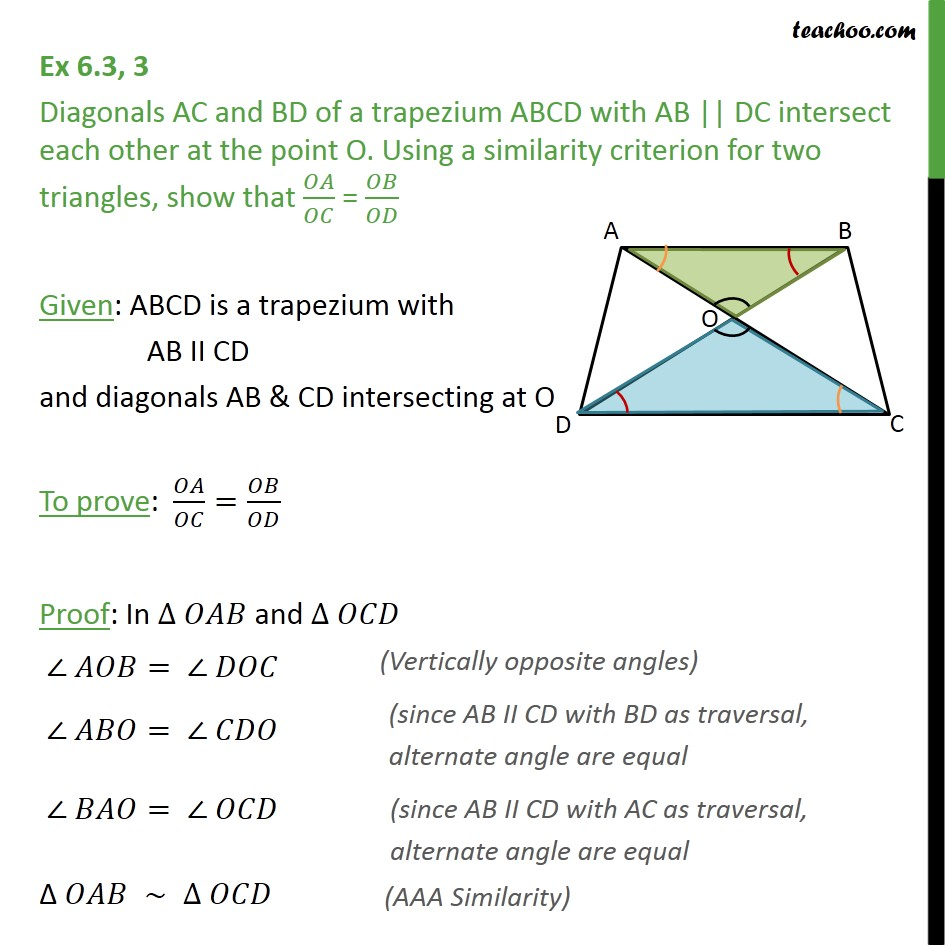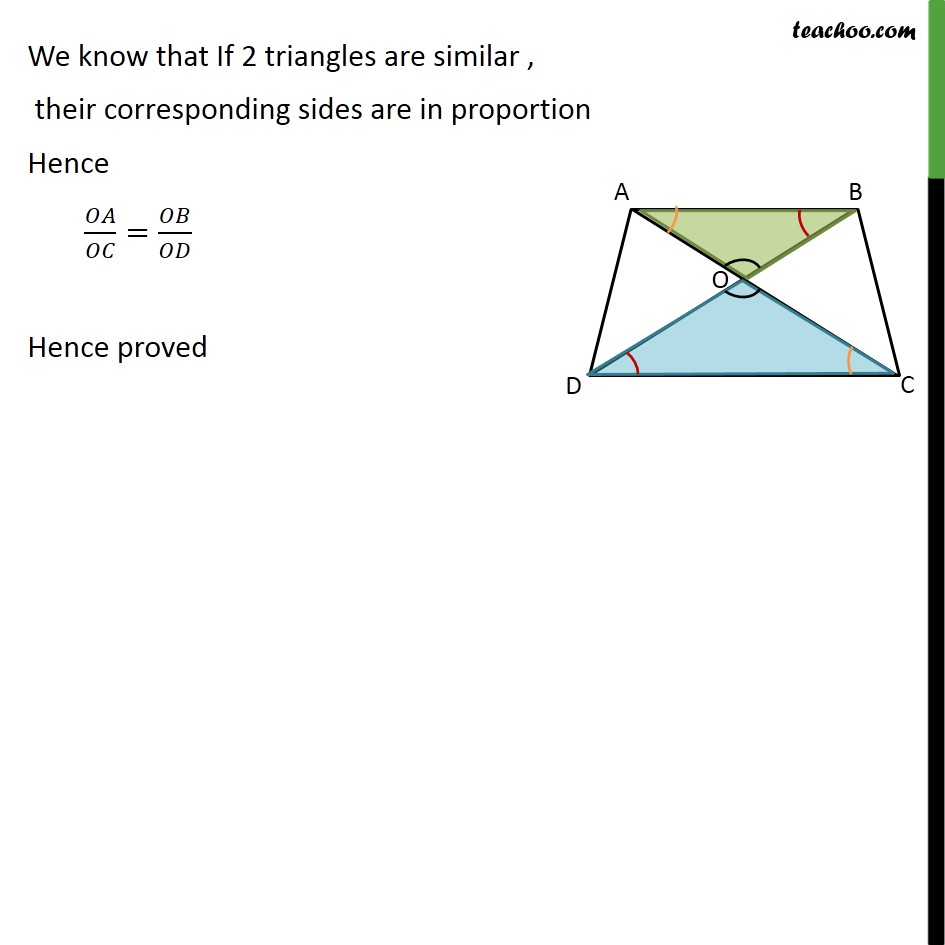Subscribe to our Youtube Channel - https://you.tube/teachoo

1. Chapter 6 Class 10 Triangles
2. Concept wise
3. AA Similarity

Transcript

Ex 6.3, 3 Diagonals AC and BD of a trapezium ABCD with AB || DC intersect each other at the point O. Using a similarity criterion for two triangles, show that / = / Given: ABCD is a trapezium with AB II CD and diagonals AB & CD intersecting at O To prove: / = / Proof: In and = = = We know that If 2 triangles are similar , their corresponding sides are in proportion Hence / = / Hence proved

AA Similarity